# Tree Tutorial 4: Predicate Logic Trees

Logical System

2013

### Skills to be acquired in this tutorial:

To become familiar with the new rules for predicate logic trees.

Colin Howson,  Logic with trees Chapter 6

### Tutorial:

#### Introduction

There are further rules for predicate logic trees (which we will come to shortly).

There is one crucial feature or property that predicate logic trees have. One of the rules, Universal Decomposition, can be used over and over again (with our conventions, it is not ticked and not made 'dead'). This means that it is possible for a branch (and a tree) to grow indefinitely. And, in turn, this has repercussions on testing for validity, satisfiability etc. If a tree is closed, the root formulas are not satisfiable (so, if the root formulas are the premises of an argument, and the negation of its conclusion, that argument is valid). But, if the tree has an open branch, matters become much more subtle. If that branch is complete, and does not contain a Universally quantified formula, the root formulas are satisfiable. But if that branch is close to complete, and does contain a Universally quantified formula, it may be possible to judge that the branch will never close or, alternatively, it may happen that the branch can be grown and grown without it becoming clear whether it will close or not.

With propositional logic trees, the tree method was 'decidable'. It was a mechanical method, that would yield, in a finite number of steps, answers to questions of satisfiability and validity.

With predicate logic trees, the tree method is undecidable. If some formulas are unsatisfiable, a tree for them will close (though, and this is important, it may be arbitrarily large). If some formulas are satisfiable, a tree for them may produce an open branch which cannot be extended, or it may produce an open branch which can be extended indefinitely. Turning this around, if you are growing a tree and it is getting bigger and bigger, you don't know whether to keep growing it in the hope that it will close shortly or to give up and conclude that the root formulas are unsatisfiable. You can be in a position where you don't know whether or not the root formulas are satisfiable

There is no mechanical method.

#### The Predicate Logic Rules

With the propositional rules, the rules themselves were motivated by truth-tables and considered what was needed to 'picture' the truth of the formula being extended. It is possible to use a similar approach for predicate logic (although, of course, there are no truth tables in predicate logic).

For an existentially quantified formula, say ∃xF(x), to be true, something needs to be F, perhaps 'a' is F ie F(a) is true. But we need to be careful here. And what we need to be careful of is whether the individual, or constant that represents it, is already in the tree or in the context. If it is already known that, for example G(a), or ¬F(a) we cannot go from {G(a),¬F(a), ∃xF(x)} (which is perfectly good and satisfiable) to {G(a),¬F(a), F(a)} which is not satisfiable (and also tells us that there is some one thing which is both G and F, which is a piece of information not in the original formulas). To avoid this problem, we need to use a completely new constant. Thus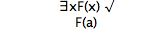where 'a' is a constant new to the branch. With the software, you do not have to choose the new constant, the computer will do it for you. For example, if 'a' occurred, the software would choose something else. Thus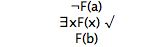Here the software has chosen 'b', to avoid the 'a' which already occurs. The software will do this for you but, of course, if you are drawing trees yourself, on a piece of paper, you need to choose a new constant when using this rule.

For a universally quantified formula, say ∀xF(x), to be true, everything needs to be F (ie F(a), F(b), F(c), ... etc., continued indefinitely, all need to be true). We cannot put infinitely many formulas into the tree at once. Instead, we put just one of them in, but allow the rule to the used again, if needed, to put another one in, and so on. Here is the rule being used 3 times in a row.

One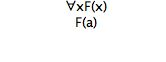Two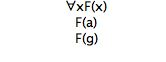ThreeNotice that the universal quantified formula is not dead, and not ticked, and this allows it to be used again and again.

The User gets to choose which instantiating constant is used. And here the advice is: (first) use constants that are already in the branch. Remember: the goal is to close the branches, so you are hoping that the universal formula will give you a formula that will contradict what you have. For example,If you choose 'b' or 'c' or 'd' as the instantiating constant, you are just wasting time and that strategy will not close the branch. You need to choose 'a'. ThusAt this point in the account of predicate trees, more can be said about whether open branches will close and the earlier remark 'it may be possible to judge that the branch will never close' . It can be proved about open branches that a) if all the formulas in it, except universally quantified formulas, that can be extended have been extended, b) that all the universally quantified formulas in it have been extended (ie instantiated) at least once (eg to a constant, say b, or to a closed term, say f(a)), and c) that all the universally quantified formulas in it have been extended (ie instantiated) for every constant or closed term that appear in that branch, that open branch will never close.

This motivates an extension to the acccount of a 'complete open' branch. If (a), (b) and (c) above are met, the branch is both complete and open.

Let us illustrate what this metatheorem and extended definition establish.

The branch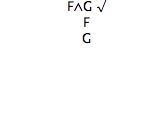satisfies (a), (b), and (c) ((b) and (c) trivially because there are no universally quantified formulas in it). It is complete and open.

The branch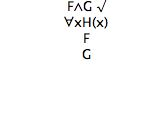satisfies (a), but not (b), because there is a universally quantified formulas in it that has not been instantiated. It is NOT complete and open.

The branch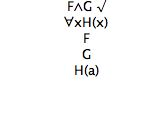satisfies (a), (b), and (c). It is complete and open.

The branchsatisfies (a), (b), but not (c). The Universal Quantifier has been instantiated but not to every constant and closed term in the branch. It is NOT complete and open.

The branch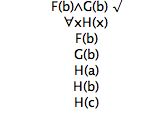satisfies (a), (b),and (c). It is complete and open. [The instantiations to H(a) and H(c) are a waste, but the branch still satisfies the definition.]

This extended definition of 'complete open branch' feeds in to the earlier results about trees

• if the tree is closed, the root formulas are not (simultaneously) satisfiable
• if a tree has a complete open branch the root formulas are (simultaneously) satisfiable
• if a tree is neither closed nor has a complete open branch, it is unknown, on the basis of that tree, whether or not the root formulas are (simultaneously) satisfiable.

There are further rules for the negations of quantified formulas, but these make a simple transformation into cases that are covered by the above two rules.

The negation of an existential formula is extended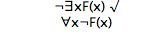ie 'not exists' becomes 'all not'.

The negation of a universal formula is extended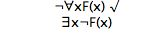ie 'not all' becomes 'exists not'.

#### Strategy

The aim is to close branches (and thus trees). It is better for this to instantiate existential quantified formulas first, giving you constants, then instantiate universally quantified formulas using the constants already in the branch

#### Reading off the (refuting) Interpretation

In the propositional logic, we used an open branch, in a complete open tree, to generate an assignment of truth values that would satisfy the initial formulas of the tree. We ran up the open branch assigning atomic formulas True and negations of atomic formulas True also (ie assigning the atomic subformula of a negation False). So, for example, if the open branch contained {¬A,B,¬C} then the assignment we were looking for was {A=False, B=True, C=False}.

This technique extends in a natural way to predicate logic. This time, we run up the open branch and i) any constant that appears there becomes an 'object' in the Universe, and ii) we make the extensions of the predicates in the branch exactly those require to make any atomic formulas True and negations of atomic formulas True also (ie making the atomic subformula of a negation False).

An example will clarify this. Take the argument

∀x(A(x)→B(x)), ∀x(¬A(x)→C(x))∴ ∀x(¬B(x)→¬C(x))

It has the tree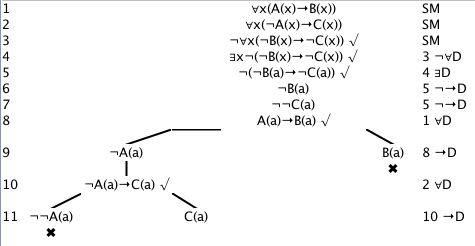and this has an open branch, which we can identify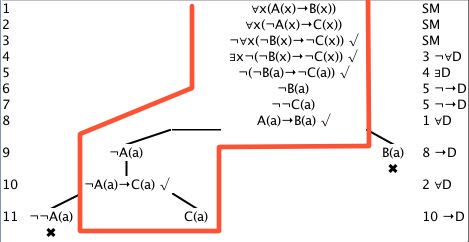In this open branch, there appears one constant, namely the constant 'a'. So the interpretation we are looking for starts

Universe = {a}

Then we need to look for the atomic formulas and negations of atomic formulas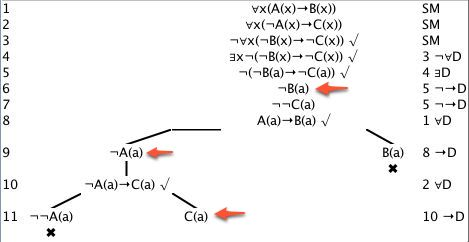And we need to get these so that Aa is False, Ba is False, and Ca is True. So what we want is

Universe = {a}
A = {}
B = {}
C = {a}

Under this Interpretation, all the initial formulas will be true (indeed, all the formulas in the branch will be true). Let us check for the initial formulas.

∀x(A(x)→B(x)) is true because nothing is A so the antecedent of the conditional ie A(x) is always false

∀x(¬A(x)→C(x)) is true (¬A(a)→C(a)) is true and the object 'a' is the only thing in the Universe so 'all of them are'

¬∀x(¬B(x)→~C(x)) is true because this is the same as ∃x(¬B(x)∧C(x)) and (¬B(a)∧C(a)) is true

Now, this tree was a tree for the argument (∀x)(A(x)→B(x)), (∀x)(¬A(x)→C(x))∴ (∀x)(¬B(x)→¬C(x)) (we wrote the premises and the negation of the conclusion to start the tree). So this argument is invalid and the Interpretation

Universe = {a}
A = {}
B = {}
C = {a}

is a 'refuting' Interpretation. Under it, the premises come out to be true and the conclusion false.

#### A small difference in syntax to the Howson book.

Howson  uses the letters 'a..w' as constants and 'x,y,z' as variables. (Then he puts subscripts on them to get infinitely many, which is what you want for proving various metatheorems.) Just to fit in with other running programs on this site, we have the smallest of changes to this. We'll use 'a..v' as constants, and 'w..z' as variables (and you can have subscripts, if you wish) y3 is a variable and c1 a constant. This difference can make a difference in limit cases; for example, our software will read w(x) as being ill-formed, because it is trying to apply a variable to a variable, whereas as Howson-style could see it as making sense and being the application of the function w to the argument x.

From a software point of view, subscripts bring in their own problems. Usually subscripts (and superscripts) are 'visual fakes'-- they are ordinary characters made a little smaller then moved down or up. (That is what was done in the previous paragraph.) But the logic software is unaware of this (just as it is unaware of your using italic, or bold, or large fonts). The logic software needs real subscripts. Here they are ₁ ₂ ₃ ₄ ₅ ₆ ₇ ₈ ₉ . Whenever the context suggests that subscripts might help, we'll supply them in a palette.

## Exercises to accompany Tree Tutorial 4

### Exercise 1 (of 3):

Determine whether these arguments are valid (ie try to produce closed trees for them)

a) ∀x(F(x)→G(x)), ∃x¬G(x) ∴ ∃x¬F(x)
b) ∀x(F(x)→∀yG(y)), F(a) ∴ ∀xG(x)
c) ∀x(A(x)→B(x)), ∀x(¬A(x)→C(x))∴ ∀x(¬B(x)→¬C(x))
d) ∃xF(x),∀x(¬G(x)→¬F(x)),∀xM(x) ∴ ∃xG(x)∧∃xM(x)

### Exercise 2 (of 3):

Determine whether these arguments are valid (ie try to produce closed trees for them)

e) ∃x(F(x)∧∀y(G(y)→L(x,y))), ∀x(F(x)→∀y(M(y)→¬L(x,y))) ∴ ∀x(G(x)→¬M(x))
f) ∀x(A(x)→F(x)), ∃xF(x)→¬∃yG(y) ∴ ∀x(∃yA(y)→¬G(x))
g) ∃x(A(x)∧¬B(x)), ∃x(A(x)∧¬C(x)),∃x(¬B(x)∧D(x))∴ ∃x(A(x)∧(¬B(x)∧D(x)))